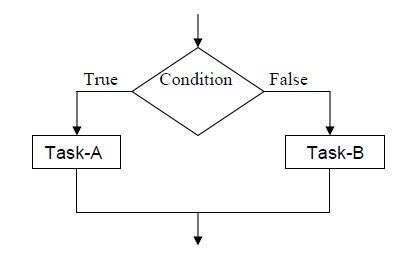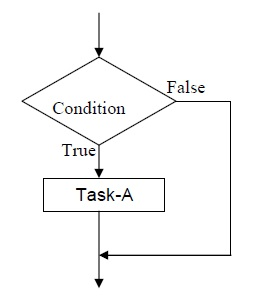# Decision Structure or Selection Structure

The decision structure or mostly commonly known as a selection structure, is case where in the algorithm, one has to make a choice of two alternatives by making decision depending on a given condition.

Selection structures are also called case selection structures when there are two or more alternatives to choose from. This structure can be illustrated in a flowchart as follows:If condition is true
else

In this example, the condition is evaluated, if the condition is true Task-A is evaluated and if it is false, then Task-B is executed. A variation of the construct of the above figure is shown below.If condition is true then

In this case, if condition is false, nothing happens. Otherwise Task-A is executed.

The selection requires the following.

• Choose alternative actions as a result of testing a logical condition
• Produce code to test a sequence of logical tests